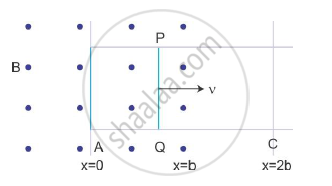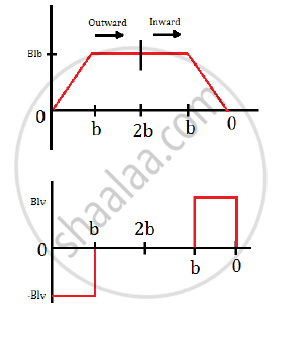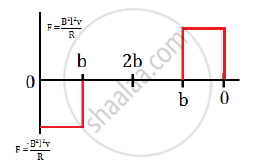# Sketch the Change in Flux, Emf and Force - Physics

Sketch the change in flux, emf and force when a conducting rod PQ of resistance R and length l moves freely to and fro between A and C with speed v on a rectangular conductor placed in uniform magnetic field as shown in the figure#### Solution

The flux enclosed by the rod is

Φ = Blx        for 0 ≤ x ≤ b

= Blx           for b ≤ x ≤ 2b

Now, the magnitude of induced emf is

epsilon=(dphi)/dt=-Bl(dx)/dt= -Blv            for 0 ≤ x ≤ b

=-Bl(db)/dt=0                                  for b ≤ x ≤ 2b

Now, the magnitude of induced current when induced emf is non-zero is ε = IR

:.I=epsilon/r=(Blv)/r

The force required to keep the conductor in motion is F =  BIl

:.F=B(Blv)/Rl=(B^2l^2v)/R

:.F=(B^2l^2v)/R                      for b ≤ x < b

= 0                          for b ≤ x ≤ 2b

Therefore, the variation of flux, emf and force isConcept: Force on a Current - Carrying Conductor in a Uniform Magnetic Field
Is there an error in this question or solution?